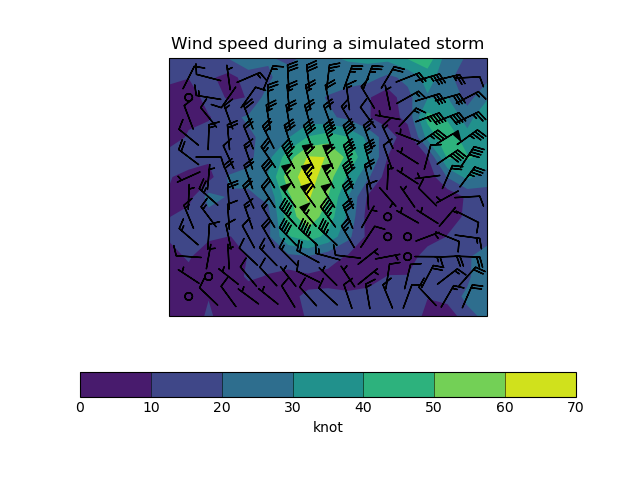# Plotting Wind Direction Using Barbs#

This example demonstrates using barbs to plot wind speed contours and wind direction barbs from wind vector component input data. The vector components are co-located in space in this case.

The magnitude of the wind in the original data is low and so doesn’t illustrate the full range of barbs. The wind is scaled to simulate a storm that better illustrates the range of barbs that are available.```import matplotlib.pyplot as plt

import iris
import iris.plot as iplt
import iris.quickplot as qplt

def main():
# Load the u and v components of wind from a pp file
infile = iris.sample_data_path("wind_speed_lake_victoria.pp")

uwind.convert_units("knot")
vwind.convert_units("knot")

# To illustrate the full range of barbs, scale the wind speed up to pretend
# that a storm is passing over
magnitude = (uwind**2 + vwind**2) ** 0.5
magnitude.convert_units("knot")
max_speed = magnitude.collapsed(
("latitude", "longitude"), iris.analysis.MAX
).data
max_desired = 65

uwind = uwind / max_speed * max_desired
vwind = vwind / max_speed * max_desired

# Create a cube containing the wind speed
windspeed = (uwind**2 + vwind**2) ** 0.5
windspeed.rename("windspeed")
windspeed.convert_units("knot")

plt.figure()

# Plot the wind speed as a contour plot
qplt.contourf(windspeed)

# Add wind barbs except for the outermost values which overhang the edge
# of the plot if left
iplt.barbs(uwind[1:-1, 1:-1], vwind[1:-1, 1:-1], pivot="middle", length=6)

plt.title("Wind speed during a simulated storm")
qplt.show()

if __name__ == "__main__":
main()
```

Total running time of the script: (0 minutes 0.145 seconds)

Gallery generated by Sphinx-Gallery